﻿ 吉林省城乡居民个人医疗支出比例及未来影响研究

# 吉林省城乡居民个人医疗支出比例及未来影响研究Study on the Proportion and Future Impact of Individual Medical Expenditure of Urban and Rural Residents in Jilin Province

Abstract: By calculating the per capita personal health expenditure and total health expenditure in Jilin Province in recent 7 years, this paper studies the proportion and future impact of personal medical expenditure of urban and rural residents in Jilin Province in recent years, in order to further improve China’s medical and health system and further improve China’s public health system.

1. 资料来源与方法

1.1. 资料来源

1.2. 研究方法

1.3. 统计学方法

2. 结果

2.1. 基于GM(1,1)模型的个人卫生支出占卫生总费用比例预测Table 1. Personal health expenditure accounts for the proportion of total health expenses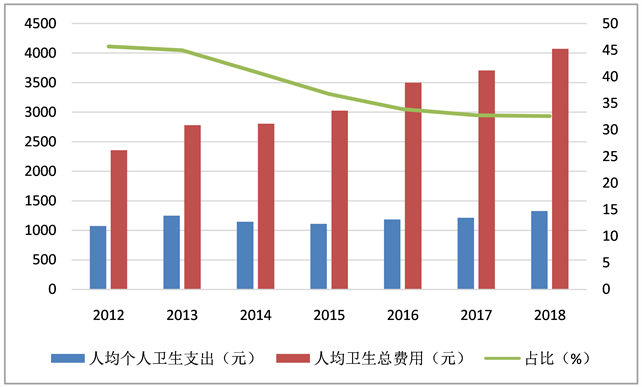Figure 1. 2012~2018 Jilin Province, urban and rural residents accounting as map

2.2. 级比检验，建模可行性分析

2.3. 灰色GM(1,1)模型的建立

${X}^{\left(1\right)}=\left({X}^{\left(1\right)}\left(1\right),{X}^{\left(1\right)}\left(2\right),\cdots ,{X}^{\left(1\right)}\left(7\right)\right)=\left(1076.63,2327.53,3475.49,4586.93,5773.83,6988.46,8315.83\right)$。其次，构造均值数列，即

${Z}^{\left(1\right)}=\left({Z}^{\left(1\right)}\left(2\right),\cdots ,{Z}^{\left(1\right)}\left(7\right)\right)=\left(1702.08,2901.51,4031.21,5180.38,6381.145,7652.145\right)$.

$Z=\left(\begin{array}{c}\frac{1}{2}\left[{X}^{\left(1\right)}\left(1\right)+{X}^{\left(1\right)}\left(2\right)\right]\\ \frac{1}{2}\left[{X}^{\left(1\right)}\left(2\right)+{X}^{\left(1\right)}\left(3\right)\right]\\ \frac{1}{2}\left[{X}^{\left(1\right)}\left(3\right)+{X}^{\left(1\right)}\left(4\right)\right]\\ \frac{1}{2}\left[{X}^{\left(1\right)}\left(4\right)+{X}^{\left(1\right)}\left(5\right)\right]\\ \frac{1}{2}\left[{X}^{\left(1\right)}\left(5\right)+{X}^{\left(1\right)}\left(6\right)\right]\\ \frac{1}{2}\left[{X}^{\left(1\right)}\left(6\right)+{X}^{\left(1\right)}\left(7\right)\right]\end{array}\right)=\left(\begin{array}{c}1702.08\\ 2901.51\\ 4031.21\\ 5180.38\\ 6381.145\\ 7652.145\end{array}\right)$

$Y=\left(\begin{array}{c}{X}^{\left(0\right)}\left(2\right)\\ {X}^{\left(0\right)}\left(3\right)\\ {X}^{\left(0\right)}\left(4\right)\\ {X}^{\left(0\right)}\left(5\right)\\ {X}^{\left(0\right)}\left(6\right)\\ {X}^{\left(0\right)}\left(7\right)\end{array}\right)=\left(\begin{array}{c}1250.90\\ 1147.96\\ 1111.44\\ 1186.90\\ 1214.63\\ 1327.37\end{array}\right)$

$B=\left(\begin{array}{c}-{Z}^{\left(1\right)}\left(2\right)\\ -{Z}^{\left(1\right)}\left(3\right)\\ -{Z}^{\left(1\right)}\left(4\right)\\ -{Z}^{\left(1\right)}\left(5\right)\\ -{Z}^{\left(1\right)}\left(6\right)\\ -{Z}^{\left(1\right)}\left(7\right)\end{array}\right)=\left(\begin{array}{cc}-1702.08& 1\\ -2901.51& 1\\ -4031.21& 1\\ -5180.38& 1\\ -6381.145& 1\\ -7652.145& 1\end{array}\right)$

2.4. 模型的拟合检验

GM(1,1)模型需要精度合适才能用以对未来的预测。通常，为了检验预测模型是不是可靠，常用后验差比值法。Table 2. Model accuracy level evaluation standard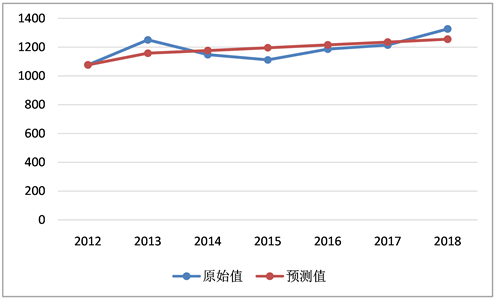Figure 2. Per capita personal health expenditure fitting effect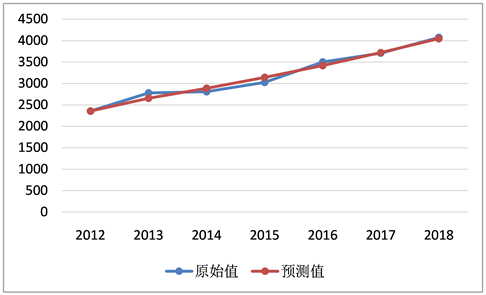Figure 3. Effectiveness of total per capital health expenses

2.5. 对2019年~2023年吉林省人均个人卫生支出进行预测Table 3. People’s personal health expenditure and its total cost proportion of health expenses in 2019~2023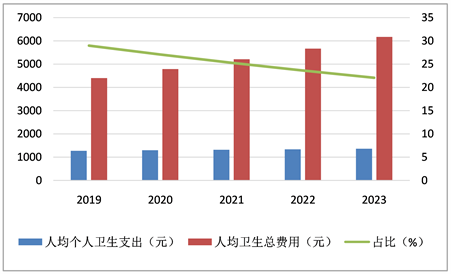Figure 4. Per capita personal health expenditure and accounting map of Jilin Province 2019~2023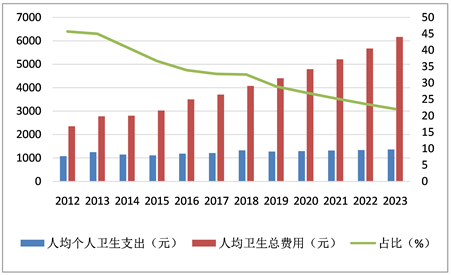Figure 5. Per capita personal health expenditure and accounting map of Jilin Province, 2012~2023

3. 讨论与结论

2012年~2018年，吉林省人均个人卫生费用从1076.63元增长到了1362.31元  。由此可见，当地的经济发展在逐步提高，同时人均总收入也在不断增加，进而带动当地的需求也逐步增长，而医疗保健费用的增加，一定程度上可以提高总体健康水平 ；另一方面居民个人医疗费用过快增长，尤其对医疗卫生资源相对薄弱的广大农村居民个人而言，不仅不利于其健康水平的进一步提高，反而会加重其经济负担，导致治病较贵的出现，甚至会加大农村居民“因病致贫，因病返贫”的风险概率 。因此，在医疗费用上，政府需要采取政策进一步稳定医疗支出费用，从各个方面去降低患者在医药上的支出。

 丁海峰, 高凯, 姜茂敏. 基于灰色GM(1,1)模型的吉林省卫生总费用预测研究[J]. 医学与社会, 2020, 33(6): 42-46.

 耿新丽, 张云霞. 吉林省卫生总费用的结构分析[J]. 财经界(学术版), 2018, 23(25): 40-41.

 洪媛媛. 广东省卫生总费用影响因素主成分回归分析[J]. 卫生软科学, 2015, 29(12): 756-759.

 颜康康, 淮明生. 灰色GM(1,1)模型在我国医疗费用预测研究中的应用[J]. 医学与社会, 2018, 31(8): 37-39.

 相静, 孔杨, 徐天和. 基于灰色系统GM(1,1)模型的山东省卫生总费用预测研究[J]. 中国卫生统计, 2016, 33(4): 653-656.

 翟铁民, 张毓辉, 万泉, 等. “十三五”以来全国及各地区个人卫生支出分析[J]. 中国卫生经济, 2020, 39(6): 13-17.

 高建民, 张文, 杨进. 陕西省卫生总费用筹资水平及结构研究[J]. 中国卫生经济, 2011, 30(5): 19-21

 高悦. DRGs助力绩效管理[J]. 中国医院院长, 2013(19): 58-59.

Top